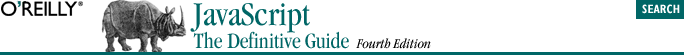home | O'Reilly's CD bookshelfs | FreeBSD | Linux | Cisco | Cisco Exam## 8.3. Methods

`o.m = f; `

Having defined the method m( ) of the object o, we invoke it like this:

`o.m( );`

Or, if m( ) expects two arguments, we might invoke it like this:

`o.m(x, x+2); `

The discussion of the this keyword should begin to clarify why we use methods at all. Any function that is used as a method is effectively passed an extra argument -- the object through which it is invoked. Typically, a method performs some sort of operation on that object, so the method invocation syntax is a particularly elegant way to express the fact that a function is operating on an object. Compare the following two lines of code:

```rect.setSize(width, height);
setRectSize(rect, width, height); ```

These two lines may perform exactly the same operation on the object rect, but the method invocation syntax in the first line more clearly indicates the idea that it is the object rect that is the primary focus, or target, of the operation. (If the first line does not seem a more natural syntax to you, you are probably new to object-oriented programming. With a little experience, you will learn to love it!)

The typical usage of methods is more clearly illustrated through an example. Example 8-2 returns to the Rectangle objects of Example 8-1 and shows how a method that operates on Rectangle objects can be defined and invoked.

#### Example 8-2. Defining and invoking a method

```// This function uses the this keyword, so it doesn't make sense to
// invoke it by itself; it needs instead to be made a method of some
// object that has "width" and "height" properties defined.
function compute_area( )
{
return this.width * this.height;
}

// Create a new Rectangle object, using the constructor defined earlier.
var page = new Rectangle(8.5, 11);

// Define a method by assigning the function to a property of the object.
page.area = compute_area;

// Invoke the new method like this:
var a = page.area( );    // a = 8.5*11 = 93.5```

One shortcoming is evident in Example 8-2: before you can invoke the area( ) method for the rect object, you must assign that method to a property of the object. While we can invoke the area( ) method on the particular object named page, we can't invoke it on any other Rectangle objects without first assigning the method to them. This quickly becomes tedious. Example 8-3 defines some additional Rectangle methods and shows how they can automatically be assigned to all Rectangle objects with a constructor function.

#### Example 8-3. Defining methods in a constructor

```// First, define some functions that will be used as methods.
function Rectangle_area( ) { return this.width * this.height; }
function Rectangle_perimeter( ) { return 2*this.width + 2*this.height; }
function Rectangle_set_size(w,h) { this.width = w; this.height = h; }
function Rectangle_enlarge( ) { this.width *= 2; this.height *= 2; }
function Rectangle_shrink( ) { this.width /= 2; this.height /= 2; }

// Then define a constructor method for our Rectangle objects.
// The constructor initializes properties and also assigns methods.
function Rectangle(w, h)
{
// Initialize object properties.
this.width = w;
this.height = h;

// Define methods for the object.
this.area = Rectangle_area;
this.perimeter = Rectangle_perimeter;
this.set_size = Rectangle_set_size;
this.enlarge = Rectangle_enlarge;
this.shrink = Rectangle_shrink;
}
// Now, when we create a rectangle, we can immediately invoke methods on it:
var r = new Rectangle(2,2);
var a = r.area( );
r.enlarge( );
var p = r.perimeter( );```

The technique shown in Example 8-3 also has a shortcoming. In this example, the Rectangle( ) constructor sets seven properties of each and every Rectangle object it initializes, even though five of those properties have constant values that are the same for every rectangle. Each property takes up memory space; by adding methods to our Rectangle class, we've more than tripled the memory requirements of each Rectangle object. Fortunately, JavaScript has a solution to this problem: it allows an object to inherit properties from a prototype object. The next section describes this technique in detail.